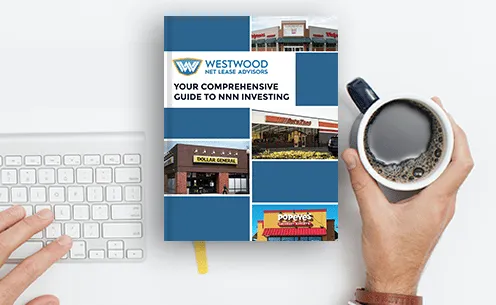# 9 Essential Performance Measurements For Commercial Real Estate

Dec 19, 2016

There are several ways of evaluating a property’s performance, but the best way to start is by taking a good look at the numbers. Below are 9 different performance measurements that will give you a good idea of whether or not to pass on a potential investment property.

## The Investment Property Performance Is Relative

The first thing to understand about measuring a performance of a commercial real estate property is that it is relative.

Two properties might have very different cap rates, for example, even if they are in the same general area. Gross income will be different, debt service will vary; all of these will result in two numbers that are very different.

A wise investor will, therefore, look at several properties in varying locations in order to evaluate how well investment property is performing.

## 1. Net Income Return On Investment

The net income of the investment property is the amount of money left after all operating expenses are deducted.To calculate it, simply take the property’s gross operating income and deduct operating expenses; the resulting number is what the return on the property would be if it were purchased in cash, without deducting capital recovery or income taxes.

## 2. Cash Return On Investment

Cash return also referred to as the cash yield or equity dividend, the rate is a ratio that gives you a good idea of what the yearly cash flow of the investment property will be.

It doesn’t take into account income taxes, changes in property value, or reductions in loan principal, however, it can be used to compare various leverage scenarios.To calculate the cash on cash return for the investment property, divide the annual cash flow before taxes by the total amount of cash invested in the property. Multiply the resulting number by 100%, and you’ll have the cash on cash return.

## 3. Total Return On Investment

The total return on investment or ROI is similar to the cash on cash return, except it takes into account the reduction in a loan that occurs when loan payments are made.

ROI is calculated by taking the expected net income (estimated expenses subtracted from estimated gross income) and dividing it by the purchase and improvement costs.This number will give you a percentage, which is the amount of money you can expect annually in relation to your initial investment. However, the answer can vary from year to year, depending on whether you invest some of the profits in a renovation.

## 4. Net Operating Income

Net operating income is calculated by deducting the amount spent on operating expenses from the gross income. This number will also tell you how much money is left to pay debt service, whether from a mortgage or private loan.NOI is also used to estimate the sales price of an investment property by multiplying the cap rate by the NOI.

## 5. Capitalization Ratio

The capitalization ratio commonly referred to as the cap rate is the ratio of the NOI to the purchase price of the investment property.

The lower the cap rate, the higher the cost of the property. However, a “good” cap rate is relative, since it depends on how much cash flow you’ll get from the property in exchange for the amount of risk you will undertake.

## 6. Debt Service Coverage Ratio

The DSCR is especially important to lenders, as it helps them determine whether or not the investment property will make enough of a profit to pay back the loan.

It is calculated by taking the NOI and dividing it by the combined total of the loan principal plus interest.Changing the loan repayment period will affect the DSCR by lowering the monthly loan payments, thereby increasing the DCSR. Most lenders will insist on a DSCR of 1.25x.

## 7. Operating Ratio

The operating ratio gives investors an idea of how well a property is being managed.

Commercial income properties have a lower OR ratio, with the exception being multifamily properties which vary depending on how much of the expense is passed on to tenants. On the other hand, triple net properties have a very low ratio since tenants pay all operating expenses and nearly all maintenance expenses.

## 8. Gross Rent Multiplier

The gross rent multiplier is similar to the cap rate. However, while the cap rate compares net income to price, the GRM compares gross income to price.

The GRM is calculated by taking the purchase price and dividing it by the gross income. This equation is actually the inverse of the equation used to calculate cap rate.

So while a higher cap rate is preferable to a lower cap rate, a lower GRM is better than a higher GRM.

## 9. Break-Even Ratio

The break-even ratio is exactly what the name implies: it tells you the amount of money the property needs to generate in order to break even. Thus the amount of money going out of the property will equal the amount of money coming in.

As the break-even ratio’s name implies, the break-even point is the point at which the total cash inflows are exactly equal to the total cash outflows. A ratio of more than 1 means there is a negative cash flow, while a ratio of less than one has a positive cash flow. It is calculated by adding the debt service to the total operating expenses and then dividing that number by the gross income.Analyzing each of these performance measurements is a good start to understanding the potential of an income property. However, keep in mind that these numbers still don’t present the entire picture: you will need to dig deeper in order to evaluate how each number relates not just to each other, but also to other comparable properties in the same location.

### Looking To Buy Commercial Property?

Find out why triple-net lease real estate investments should be part of your investment portfolio.